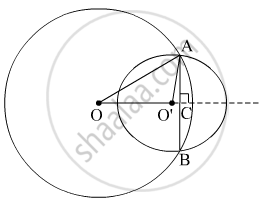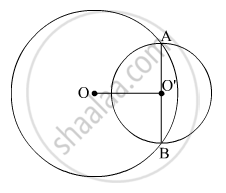# Two circles of radii 5 cm and 3 cm intersect at two points and the distance between their centres is 4 cm. Find the length of the common chord. - Mathematics

Two circles of radii 5 cm and 3 cm intersect at two points and the distance between their centres is 4 cm. Find the length of the common chord.

#### SolutionLet the radius of the circle centered at O and O' be 5 cm and 3 cm respectively.

OA = OB = 5 cm

O'A = O'B = 3 cm

OO' will be the perpendicular bisector of chord AB.

∴ AC = CB

It is given that, OO' = 4 cm

Let OC be x. Therefore, O'C will be x − 4

In ΔOAC,

OA2 = AC2 + OC2

⇒ 52 = AC2 + x2

⇒ 25 − x2 = AC2 ... (1)

In ΔO'AC,

O'A2 = AC2 + O'C2

⇒ 32 = AC2 + (x − 4)2

⇒ 9 = AC2 + x2 + 16 − 8x

⇒ AC2 = − x2 − 7 + 8x ... (2)

From equations (1) and (2), we obtain

25 − x= − x2 − 7 + 8x

8x = 32

x = 4

Therefore, the common chord will pass through the centre of the smaller circle i.e., O' and hence, it will be the diameter of the smaller circle.Length of the common chord AB = 2 O'A = (2 × 3) cm = 6 cm

Is there an error in this question or solution?
Chapter 10: Circles - Exercise 10.4 [Page 179]

#### APPEARS IN

NCERT Class 9 Maths
Chapter 10 Circles
Exercise 10.4 | Q 1 | Page 179

Share